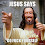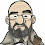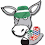## Monday, December 22, 2014

### NFL Picks Contest- Week 16 Results and Standings

Here are the Week 16 results and standings in the NFL Picks Contest. cokeboy99 ascends to the top.

Here are the current standings;

cokeboy99- 27-(8)+21+34+16+13+20+38+16+51+43+13+32+16+20+39 = 391 pts.
OneAndDone- 27+17+17+34+41+5+31+38+16+24+17+30+32+16+18+27 = 390 pts.
MOJO-  27+17+17+29+16 +24+32+37+16+34+17+30+32+16+18+12 = 374 pts.
Vegas Vic- 27+17+21+29+16+5+32-(3)+20+31+17+29+32+16+17+39 = 345 pts.
Captain Crunch- 27+17+17+29+16+5+35+38+24+24-(17)+30+32+34+17+11 = 339 pts.

Music City Momma- 13+17+21+29+16-(7)+32+38-(3)+31+17+30+32+17+17+39 = 339 pts.
TwoMinuteWarning- (3)+17+37+29+16-(7)+35+37+20+24-(17)+19+32+17+18+39 = 313 pts.
mrben09- 27+17+17+29+16+24+31+37-3+24-(15)+30+32+16+18+11 = 311 pts.
Grange95- (3)+17+21+29+16+24+11+20+16+0+43+13+62+34-(8)+16 = 311 pts.
lightning36- 27+17+17+29+16+24+31+24+20+34-(15)+30+32-(31)+18+27 = 300 pts.

ggrouchie- 27+17+17+34+41+13+20-(3)+24+24-(15)+30+32+16+18-(2) = 293 pts.
Neophyte- 27+17-(19)+0+41+24+21+0+24+24+17+14+32+24+28+0 = 274 pts.
Coach- 27+17+21+29+20+24+20-(3)+16-(7)-(20)+14+11+16+26+11 = 222 pts.

Week 16 scores

angerisagift- Dallas (W 42-7)= 45 pts.
MOJO- Miami (W 37-35)= 12 pts.
Vegas Vic- Seattle (W 35-6)= 39 pts.
Captain Crunch- New England (W 17-16)= 11 pts.
OneAndDone- Green Bay (W 20-3)= 27 pts.

mrben09- New England (W 17-16)= 11 pts.
ggrouchie- Buffalo (L 24-26)= (2) pts.
Coach- New England (W 17-16)= 11 pts.
lightning36- Green Bay (W 20-3)= 27 pts.

Neophyte- No Pick = 0 pts.
Music City Momma- Seattle (W 35-6)= 39 pts.
TwoMinuteWarning- Seattle (W 35-6)= 39 pts.
Grange95- Detroit (W 20-14) = 16 pts.
cokeboy99- Seattle (W 35-6)= 39 pts.

Thanks for playing.

Week 17 picks are due by Saturday at 6pm (CDT). Only Sunday and Monday games are eligible.

Good luck!

1.Bengals 4 my pick this week. now to finish strong so momentum carry into next season, blah blah. but thx again 4 this contest always fun. happy kwanzaa hopefully moms is doing/getting better

2.3.Merry Christmas to you and your family!

1.Thanks Dave.

Hope yours went well!